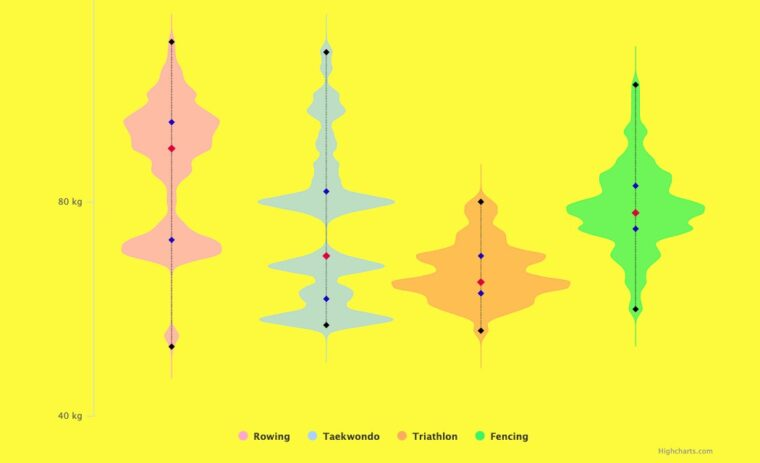# Violin PlotIn this article, we will show you how to create an interactive violin plot with Highcharts.

We will start by describing the violin plot first; then, we will walk you through the code.
The demos below display the 2012 Olympic male and female athletes’ weight of the following disciplines: taekwondo, rowing, triathlon, and fencing. The violin plot visualizes the distribution shape and the probability density (athletes’ weight) across each discipline compellingly and intuitively:

From the charts above and thanks to the violin type plot, we can easily find a similar pattern among males and females within the same discipline. Both male and female triathlon athletes’ density weight shapes show less variability compared to the other disciplines. The taekwondo athletes in both genders are widespread and have multiple clusters.

Well, you have got the picture, the violin plot is the best chart to display and compare the density distribution of a data set.

Now, it is time to explore the code part.
The code is divided into two main sections:

1. Process violin data.
2. Create the chart.

## Process violin data

The function `processViolin()` (check the GitHub link) is at the heart of the violin plot. The function is built around the kernel density estimation (KDE). Basically, a violin plot is a KDE and its negative displayed in opposition to each other. The function `processViolin()` gets the data set, in our case athletes’ weight, with a few extra parameters, then generate the density shape and measures of descriptive statistics:

```function processViolin(step, precision, densityWidth, ...args) {
…
return {
xiData,
results,
stat
};
}```

Here is the description of the function’s parameters:

• step is the minimum data set unit. The step is used to sample the data set and create the KDE.
• precision is used to refine the violin plot at the extremities and in the thin spots, the smallest this parameter is the more points you get on the extremities and the thin spots on the chart.
• densityWidth is used to widen the violin. This parameter should be equal to 1 to reflect the result of the KDE values. Nevertheless, for visibility purposes, you are free to change the `densityWidth` to get a wider and visible shape.
• args is one or many arrays that represent the data set. In our case, args is four arrays of weight athletes, one array for each discipline.

So you might wonder, how does it work in practice? Well, this is how it looks:

```let step = 1,
precision = 0.00000000001,
width = 3;
let data = processViolin(step, precision, width, rowing, taekwondo, triathlon, fencing);```

Notice the data returned by the `processViolin()`. `data` is a set of three arrays:

• xiData is the xAxis data generated using the step and the range of the athletes’ weights data.
• results include all the violin charts data.
• stat is the array with all the descriptive statistical coefficients.

Once the violin data, for each series, is generated thanks to the `processViolin()` function, the next step is to render the data.

## Create a chart

The chart creation is pretty simple to set up. The type of the series is `areasplinerange`; this chart type allows us to get the violin shape using a range. The range is the result of the calculation of both the positive and negative KDE values. The option `inverted: true` helps to get the violin chart vertical instead of horizontal:

```chart: {
type: "areasplinerange",
inverted: true,
animation: true
}```

To be sure that only the required number of the categories are displayed, be sure to restrict that number using the code below, where the min and max options have the range of the exact number of categories, in our case four: “Rowing”, “Taekwondo”, “Triathlon”, and “Fencing”.

``` yAxis: {
..
min: 0,
max: data.results.length - 1,
...
},```

One last trick to get the right violin shape is to get rid of the marker. Otherwise, you will end up with symbols all around the external lines of each series:

```plotOptions: {
series: {
marker: {
enabled: false
},
...
},```

Up to this point, the violin charts look great. Nevertheless, for more clarity, we can add some descriptive statistical coefficients such as the median (red dot), max (blue dot), min (blue dot), first quartile (black dot), and the third quartile (black dot):

The good news is all the data we need to achieve the chart above are already available in the stat array. All we have to do is to structure the information then render it using a line chart:

```stat.forEach((e, i) => {
statData.push([]);
statData[i].push(
{ x: stat[i], y: i, name: "Min", marker: { fillColor: mColor } },
{
y: i,
x: stat[i],
name: "Q1",
marker: { fillColor: qColor, radius: 4 }
},
{
y: i,
x: stat[i],
name: "Median",
marker: { fillColor: medianColor, radius: 5 }
},
{
y: i,
x: stat[i],
name: "Q3",
marker: { fillColor: qColor, radius: 4 }
},
{ y: i, x: stat[i], name: "Max", marker: { fillColor: mColor } }
);
});
let statCoef = [];
for (col = 0; col < 5; col++) {
statCoef.push([]);
for (line = 0; line < chartsNbr; line++) {
statCoef[col].push([(x = stat[line][col]), (y = line)]);
}
}```

The violin chart is a handy tool to visualize the data distribution and the probability density. We encourage you to use the violin chart type in your projects besides the histogram and the box plot charts, as each one of those chart types reveals a piece of your data secrets.